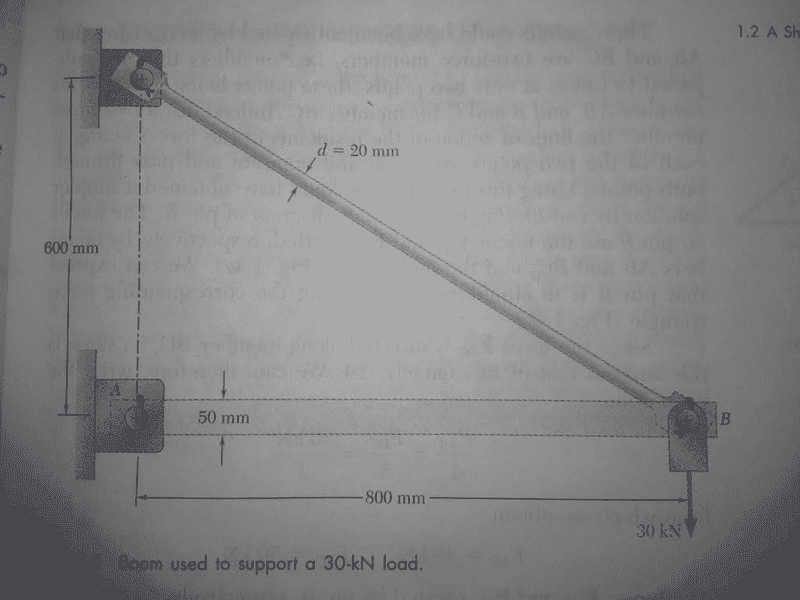# What am I doing wrong on this Statics Problem?

• k_squared

## Homework Statement

I signed up for Mechanics of Materials, but it turns out my statics is very rusty. Here is the problem, we are to compute the six component forces about the joints:## Homework Equations

Force and moment equations are the name of the game.

## The Attempt at a Solution

[/B]
My problem arises thusly:

I sum the moment about A. I place: C_x(.6)+(B_y).8=0. We know from the diagram that b_yu = 30. Simply solve this equation, and the result is C_x = 40. This is correct, according to the book.

Now, I go to sum the moments about B, C_x(.6)+A_y(.8)=0. This yeilds A_y =30. According to the book A_u=0. What is invalid about my approach?

Thanks a ton.

Your equation assumes C_y is zero.

Chet